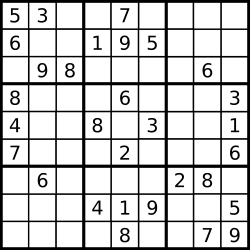## C# Sudoku solver source codeFor a while, I’ve wanted to build a little C# library to solve Sudoku puzzles. Something that would take the known values and figure out the values for the empty squares.

Since I had some time today, I went ahead and wrote it. Here it is, for any of you who want to use it.

General library information

For the 9 by 9 grid, I’ve numbered the rows and columns from 1 to 9. For the rows, 1 is the top row, 9 is the bottom. For the columns, 1 is the leftmost column, 9 is the rightmost column.

The Sudoku solver classes

This library only consists of two classes – the Square class (to hold information about each individual square) and the Board class (which holds the collection of Square objects and is where the UI would do most of its interaction).

I’ll also show the unit test class I created, to test if it can find all the values for the empty squares.

Square.cs

The Square class is mainly a “dumb bucket of values”. It only holds values for later retrieval and modification. The only calculation is does it to determine which 3×3 “block” a particular square is in.

When a Square object is instantiated, it sets the Row and Column property values (from the passed-in parameter values) and populates the PotentialValues list with the values from 1 through 9 (since the square could potentially be any one of those values at this point).

Notice that many of the properties have private setters, since an app should never have a reason to change those values. Also, many of the properties are “internal” – including the constructor. That’s because they should only ever be used by the Board object.

Board.cs

In the Board class’ constructor, the Squares list is populated with two small loops that give us the 81 squares (9 rows, with 9 columns).

The “brains” of this library is the SetSquareValue() method. When you call set a square’s value through this method, it will remove that value from the PotentialValues list for all other squares in the same row, column, or 3×3 block.

Then, it will see if there are any squares that don’t have a value, but only have one remaining PotentialValue. If so, it will set that square’s value to its only possible value, using the same method. This is a “recursive function” – it can possibly end up calling itself for another square in the list.

This happens every time you set a square. So, there is no “Solve()” method. The puzzle automatically gets solved as you add the known square values.

TestBoard.cs

This is a unit test that populates a Sudoku puzzle with values in 35 squares. Those were enough to let the program determine the values for the remaining 46 squares.

I got these values from a Sudoku game I found on the web.

At the end, you’ll see that the number of remaining unsolved squares is zero (in the Assertion at the end of the test).

Summary

This is one simple way to write some C# code that will solve a Sudoku puzzle. There are certainly other ways to write it, and this might give you an idea of how to write your own Sudoku engine.

You should be able to connect this to a UI, either in a desktop app or a web app, without too much work.